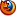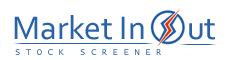Please enable JavaScript to view this page content properly Log In | Sign Up
 Pre-Tax Profit Margin Leverage Ratio Current Ratio Price / Book Ratio Price / Tangible Book Ratio Price / Cash Flow Ratio Price / Free Cash Flow Ratio Debt / Equity Ratio Price / Sales Ratio Income per Employee
 Current Ratio Action Current Ratio: More than 20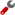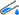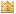Current Ratio: 10 to 20Current Ratio: 8 to 10Current Ratio: 5 to 8Current Ratio: 3 to 5Current Ratio: 2 to 3Current Ratio: 1 to 2Current Ratio: 0 to 1Best viewed in